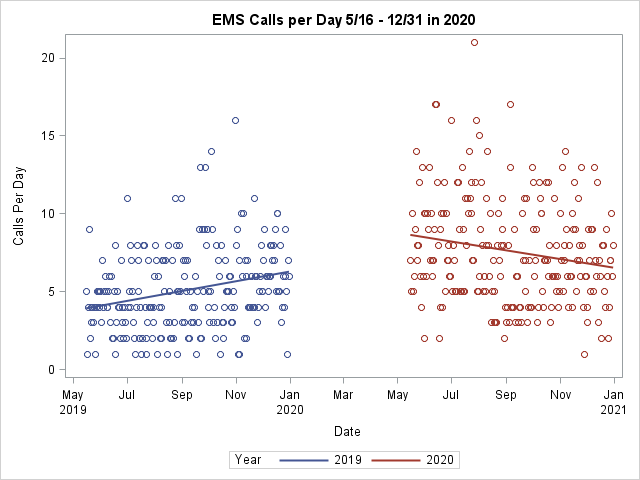## Plot Two Regression Lines on Same Scatter Plot By Year: X-Axis Date MM/DD

0
I have a scatter plot of calls / time. My x variable is supposed to be the date (Day/Month) and my Y variable is a number of calls on each date. I would like to plot two regression lines using PROC SGPLOT REG, one for 2019 and one for 2020. However, I cannot figure out a way to make my dates correspond to 1-365, which I need in order to do this.

I used a date value in SAS and changed the format to mm/dd, but this doesn't help because the regression lines are just on either end of the graph rather than overlapping (picture attached) (I realize this is because SAS dates correspond to numbers from 1/1/1960). What I want is the mm/dd to correspond to numbers 1-365 so I get two overlapping regression lines to show how the trends changed from one year to the next. Anyone know how I can do this?

Thank you so much!1 ACCEPTED SOLUTION

Accepted Solutions

## Re: Plot Two Regression Lines on Same Scatter Plot By Year: X-Axis Date MM/DD

I have had several similar projects. The approach as @WarrenKuhfeld has done is to standardize all the dates to a common year and then on the X axis use a format that does not display year.

Another method to standardize would be

date = mdy(month(date),day(date),<year>);

where Year would be the year picked as a standard date. Suggest a leap year so you don't get odd artifacts when one of your data years is leap year.

With a known standard year you could still use Xaxis values such as Values=('01MAYYYYY'd to '01JAN<year+1'd by month).

Date5. format will display ddMON, no year.

6 REPLIES 6

## Re: Plot Two Regression Lines on Same Scatter Plot By Year: X-Axis Date MM/DD

data new;

set old;

days1 = date1 + 1 - '01May2019'd;

days2 = date2 + 1 - '01May2021'd;

run;

Then plot days1 and days2. Adjust the variables and dates as necessary.

## Re: Plot Two Regression Lines on Same Scatter Plot By Year: X-Axis Date MM/DD

I have had several similar projects. The approach as @WarrenKuhfeld has done is to standardize all the dates to a common year and then on the X axis use a format that does not display year.

Another method to standardize would be

date = mdy(month(date),day(date),<year>);

where Year would be the year picked as a standard date. Suggest a leap year so you don't get odd artifacts when one of your data years is leap year.

With a known standard year you could still use Xaxis values such as Values=('01MAYYYYY'd to '01JAN<year+1'd by month).

Date5. format will display ddMON, no year.

## Re: Plot Two Regression Lines on Same Scatter Plot By Year: X-Axis Date MM/DD

This is what we do as well. Just a note that we don't standardize based on day, ie January 1 to January 1 is not what we do because our business has weekly spikes and weekend dips so we align the first Mondays in the year and then go from there. This is quite common when aligning your time series and helps to account for seasonality a bit more in the graph. It depends on your usage and data though so go what you need.

You can convert a date to a day of year using the following calculation, regardless of year.

DayOfYear = date - intnx('year', date, 0, 'b') + 1;
year = year(date);

SGPLOT

series x=dayofYear y = value / group = year;

## Re: Plot Two Regression Lines on Same Scatter Plot By Year: X-Axis Date MM/DD

This was exactly what I needed, thank you so much! I removed the +1 however, because with that all my dates were a day off. I accidently posted this in VA by mistake, I am just using SAS 9.4.

## Re: Plot Two Regression Lines on Same Scatter Plot By Year: X-Axis Date MM/DD

Are you doing this in SAS VA though? If so, that's a different story than doing it via code. You're posting these questions in VA which is a BI tool, not a programming tool?

## Re: Plot Two Regression Lines on Same Scatter Plot By Year: X-Axis Date MM/DD

Thank you so much!
Discussion stats
• 6 replies
• 422 views
• 4 likes
• 4 in conversation